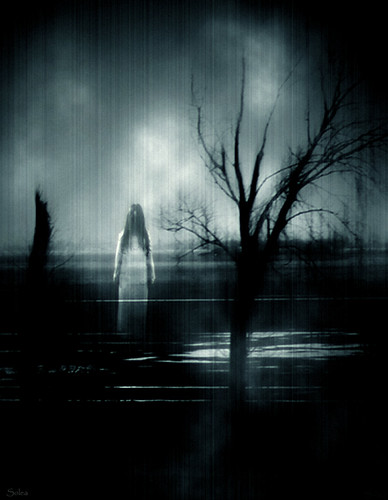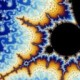## The New Post Game (The Friday Morning Remix ‘clue’ Edition)Waay too busy to write anything significant but here are some clues on what my next post is about:O.K., so who has a guest pass to xenii for me?

Speaking of walled gardens, a Turner employee examines YouTube.

Fuck The PoliceModern Day PiratesDigg vs. The New York Times

Outkast song leaks

Building A Blog Network

Explore posts in the same categories: blog networks, Digg, google, outkast, pirates, police, stephen hawkings, worry, YouTube

### 8 Comments on “The New Post Game (The Friday Morning Remix ‘clue’ Edition)”

1.TerryC Says:

drop me an email. I got a pass for you.

2.chartreuse Says:

delish.

3.jennifer Says:

The cops looting the WalMart. Incredible.
The leaked outkasts songs were good,too.
But what I want is to hang out at Xenii so I can meet a man worthy of me!

As a game, this is weak. We all know you are going to be writing about pirated videos put on YouTube. Right?

4.range Says:

I like the pirates, didn’t we all want to be pirates at some point in our lives? Goes hand in hand with Johnny Depp acting all drunk again in another pirate movie.

5. fgdrstgvd…

ceasrsv vtrtv werce v weqrqwer rwreqrwr…

6.range Says:

Shit Char, did you party too hard over the weekend?

7. hwkbnbjy Says:

iflwasnfni

8.Joe Nahhas Says:

E=mc²/2
2009 is the end of Einstein’s space-jail of time and Fraud symbol E=mc²
Joenahhas1958@yahoo.com
Time is not a structure like space to allow space-to time-back to space jumping claimed by Physicists regardless of what physicists have to say about it because Physics is a business and not necessarily science or scientific and like every business it comes with fraud and fraud is Einstein’s space-time (x, y, z, it) continuum that led to fraud symbol E=mc² and yes I am saying that 109 years of Nobel prize winners physics and physicists are all wrong and space-time physics is based on scientific fraud. When “results” expected and “No” discovery, Physicists rigged Physics for grant money since the start of the industrial revolution. Physics today is at least 51 % fraud!
r ——————>>Exp (ì w t) ———->> S=r Exp (ì wt) Nahhas’ Equation
Orbit——–>> Orbit light sensing——>> Visual Orbit; Exp = Exponential
Particle —->> light sensing of moving objects———— >> Wave
Newton———>>light sensing———->> Quantum
Quantum = Newton x Visual Effects
Quantum – Newton = Relativistic = Optical Illusions
E (Energy by definition) = mv²/2 = mc²/2; if v = c
m = mass; v= speed; c= light speed; w= angular velocity; t= time
S = r Exp (ì w t) = r [cos (wt) + ì sin (wt)] Visual effects
P = visual velocity = change of visual location
P = d S/d t = v Exp (ì w t) + ì w r Exp (ì w t)
= (v + ì w r) Exp (ì w t) = v (1 + ì) Exp (ì w t) = visual speed; v = wr
E (visual energy= what you see in lab) = m p²/2; replace v by p in E = mv²/2
= m p²/2 = m v²/2 (1 + ì) ² Exp (2ì wt)
= mv²/2 (2ì) [cosine (2wt) + ì sine (2wt)]
=ì mv² [1 – 2 sine² (wt) + 2 ì sine (wt) cosine (wt)];v = speed; c = light speed
wt = π/2
E (visual) = ìmv² (1 – 2 + 0)
E (visual) = -ì mc² ≡ mc² (absolute value;-ì = negative complex unit) If v = c
w t = π/4
E (visual) = imv² [1-1 +ỉ] =-mc²; v = c
wt =-π/4+ỉln2/2; 2ỉ wt=-ỉπ/2 – ln2
Exp (2i wt) = Exp [-ỉπ/2] Exp [ln(1/2)]=[-ỉ (1/2)]
E (visual) = imv² (-ỉ/2) =1/2mc² v = c